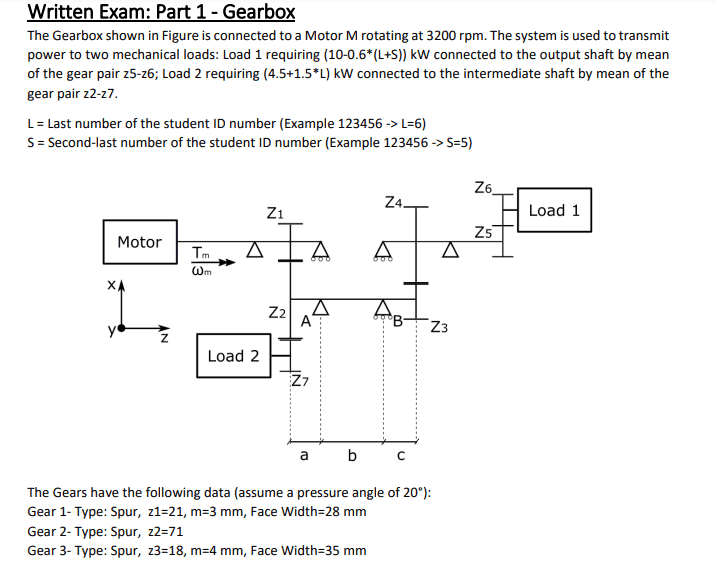# How to calculate the power distribution through this gearbox?

• Engineering
• Amaelle
In summary, the conversation discusses the issue of understanding the flow of power in a gearbox and why the power in load 2 and load 1 are not equal to the power of the motor. The solution involves finding the angular velocity and torque in all the shafts and understanding the concept of power in watts. It is also emphasized to show the attempt in solving the problem and providing the full problem statement and information. Finally, the conversation concludes with the issue being resolved.f

#### Amaelle

Homework Statement
How the power is distributed inside the gearbox
Relevant Equations
Gerbox
Greeting!My problem is to understand the flux of power inside this gearbox
for example I can´t understand why the power in load 2 and load 1 are not equal to the power of the motor (suppose 100% efficiency). if this gearbox was an energy circuit (how to know that the energy is transmitted and shared) thank you!

I can´t understand why the power in load 2 and load 1 are not equal to the power of the motor
Where did you find the power of the motor ?

Find the angular velocity of all the shafts.
Back compute the torque in all the shafts.
The product of torque and angular velocity is power.
Power in watts is the flow of energy in joules per second.
You need to show your attempt.

•berkeman
Where did you find the power of the motor ?

Find the angular velocity of all the shafts.
Back compute the torque in all the shafts.
The product of torque and angular velocity is power.
Power in watts is the flow of energy in joules per second.
You need to show your attempt.
thank you
I already have the solution, I just want to understand the intuition behind it, like for example if it was a circuit would be in parallel or in serie, I undertand know that it´s in serie and that the total power goes to the intermdiate shaft before it´s splited between load1 and load2

Why do you say ...
... for example I can´t understand why the power in load 2 and load 1 are not equal to the power of the motor (suppose 100% efficiency).
If you think the power account is not balanced, then show us what you think the powers are, and how you calculated them.

••Amaelle and Lnewqban
Can you visualize Z2-Z3 shaft as having motor power as input and L1 and L2 as two parallel outputs?
M power = L1 power + L2 power

Could you show us the whole problem statement and information?
We are missing the main question(s) and Z4 through Z7.

•Amaelle
Why do you say ...

If you think the power account is not balanced, then show us what you think the powers are, and how you calculated them.
thank you I could solve the issue :)

Can you visualize Z2-Z3 shaft as having motor power as input and L1 and L2 as two parallel outputs?
M power = L1 power + L2 power

Could you show us the whole problem statement and information?
We are missing the main question(s) and Z4 through Z7.
thank you I could solve the issue :)

•Lnewqban